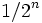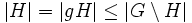# Subgroup of size more than half is whole group

DIRECT: The fact or result stated in this article has a trivial/direct/straightforward proof provided we use the correct definitions of the terms involved
View other results with direct proofs
VIEW FACTS USING THIS: directly | directly or indirectly, upto two steps | directly or indirectly, upto three steps|
This fact is an application of the following pivotal fact/result/idea: Lagrange's theorem
View other applications of Lagrange's theorem OR Read a survey article on applying Lagrange's theorem
This article is about a result whose hypothesis or conclusion has to do with the fraction of group elements or tuples of group elements satisfying a particular condition.The fraction involved in this case is 1/2
View other such statements

## Statement

In a finite group, any subgroup whose order is more than half the order of the group, must be the whole group.

## Applications

This result is used to establish that certain big subgroups of the group are actually the whole group. For instance, if we want to prove that an element is in the center of a group, it suffices to prove that its centralizer has cardinality more than half the cardinality of the group. Similarly, to show that a group is Abelian, it suffices to show that its center has cardinality more than half the cardinality of the group.

A specific application is:

### Applications to probabilistic algorithms

Probabilistic algorithms that deal with groups typically use ideas like this. In such algorithms, we need to check whether a given group$G$ equals a subgroup$H$, using an algorithm that generates a random element of$G$ and tests whether that element is in$H$. If$H = G$, then the answer is always yes, otherwise the answer may be yes or no.

The idea is that if the element is not in$H$, then with probability at least 1/2, the algorithm detects this by picking one random element. Hence, by repeating the random element test$n$ times, we can ensure that if$H$ is not the whole of$G$, the probability of getting yes all$n$ times is at most$1/2^n$. Thus, if$n$ is large enough, then we can use the random element test to say, with very high chances of accuracy, whether or not$H = G$.

This approach, and modifications of it, are used in primality testing. The crude Fermat primality test, for instance, can quickly determine, with a very high probability of correctness, whether or not a number satisfies the condition of being a (prime or absolute pseudoprime). Unfortunately, this test in its raw form cannot distinguish the primes from the absolute pseudoprimes.

More sophisticated tests, such as the Rabin-Miller primality test, use stronger versions of this idea to produce better probabilistic estimates.

## Related facts

### Generalizations to other algebraic structures

The analogous result holds for quasigroups, even though it is not true that the order of a subquasigroup divides the order of the group. The reason why the result continues to hold for quasigroups is that it is still true that the left coset of any element outside the subquasigroup is disjoint from it, and of the same size.

Further information: Subquasigroup of size more than half is whole quasigroup

### Infinite version

A closely related fact is the following, for infinite groups: in any infinite group, any proper subgroup has an infinite complement.

### Analogue with measures

The statement has an obvious analogue for a group with a left-invariant measure, and a measurable subgroup: any measurable subgroup has measure at most half the measure of the whole group.

### Facts about subgroups of large index

It is possible to have subgroups whose size is exactly half that of the group. We can say things about such subgroups. In general, small-index subgroup techniques help us say things about subgroups whose size is large relative to that of the group:

## Proof

### Proof using Lagrange's theorem

By Lagrange's theorem, the order of the subgroup must divide the order of the group. Since this is greater than half the order of the group, it must be the whole group.

### Proof using existence of an element outside the subgroup

This proof generalizes to quasigroups and to the infinite version.

Given: A finite group$G$, a proper subgroup$H$

To prove:$|H| \le |G|/2$

Proof: Pick$g \in G \setminus H$ (this can be done since$H$ is a proper subgroup of$G$). Then, the left coset$gH$ is disjoint from$H$, and has the same size as$H$. Thus:$|H| = |gH| \le |G \setminus H|$

Hence, we get:$2|H| \le |H| + |G \setminus H| = |G| \implies |H| \le |G|/2$